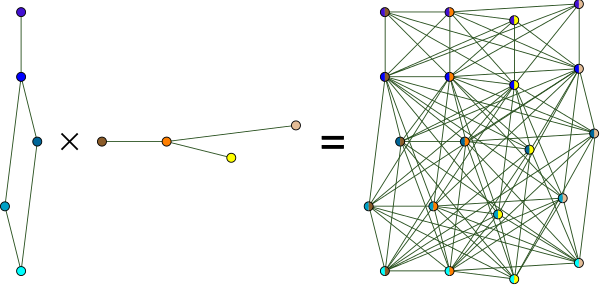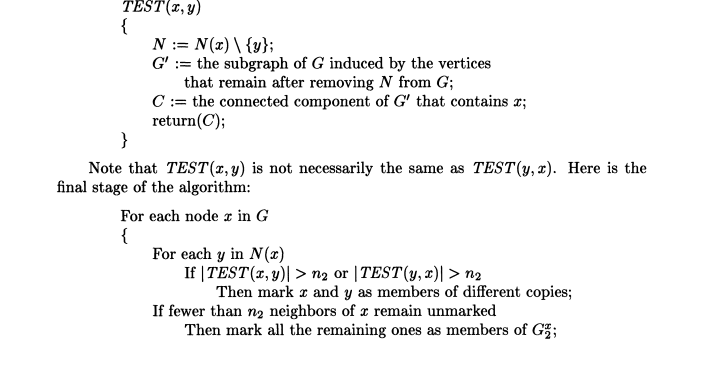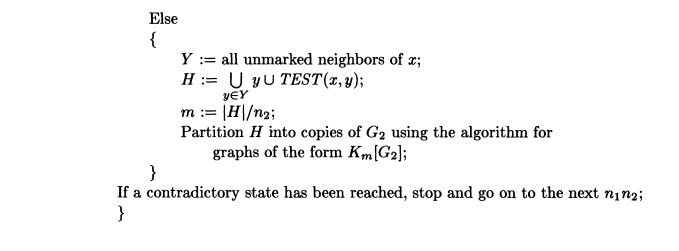# Question:An inverse problem of lexicographic product of graphs

## Question:An inverse problem of lexicographic product of graphs

Maple

In graph theory, the lexicographic product  or graph composition G ∙ H of graphs G and H is a graph such that

- the vertex set of G ∙ H is the cartesian product V(G) × V(H);
- and any two vertices (u,v) and (x,y) are adjacent in G ∙ H if and only if either u is adjacent with x in G or u = x and v is adjacent with y in H.Given two graphs, it is easy to obtain their lexicographic product. However the inverse process does not look so easy.

Recognition problem: Given a graph G, can we guess whether there exist graphs G_1,...,G_k such that G=G_1 ∙ ⋯ ∙G_k ?

I read the book "Handbook of product graphs" and wiki, that say that the recognition complexity of lexicographic products is polynomially equivalent to the graph isomorphism problem

For the lexicographic product, I know that there is some algorithm without codes to implement the decomposition of the lexicographic products of a graph.

• Feigenbaum, J.; Schäffer, A. A. (1986), "Recognizing composite graphs is equivalent to testing graph isomorphism", SIAM Journal on Computing, 15 (2): 619–627, doi:10.1137/0215045 (https://www.cs.yale.edu/homes/jf/FS-SICOMP86.pdf)

However, I did not understand the algorithm process mentioned in the article, nor did I see the program implementation of this algorithm. About 5 months ago, I asked similar questions on multiple platforms, but did not receive any feedback.The potential algorithm can help us discover some theorems, so I am very interested in the implementation of the algorithm in the above article.

PS:We also know that there are already polynomial algorithms for the decomposition of the cartesian product of a graph. A polynomial time algorithm for finding the prime factors of Cartesian-product graphs", Discrete Applied Mathematics, 12 (2): 123–138, doi:10.1016/0166-218X(85)90066-6, MR 0808453 and we can find the java codes for implementation of finding the prime factors of Cartesian-product graphs.﻿